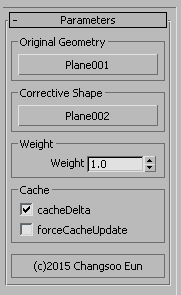# MCG: ExtractDeltas

This is a MCG modifier which extract the difference(deltas) between two meshes and let you apply the deltas to a different mesh.
You can use this modifier to make a corrective morph target. Check out this video for how to utilize this modifier.

This  modifier need two meshes to calculate deltas, “Original geometry” and “Corrective shape”.
This modifier will go through each vertex of two meshes and extract the difference(Corrective Shape- Original Geometry).
Then, the modifier will offset the vertex position with the calculated deltas.
Therefore, all three meshes must have the same number of vertex and topology.You can also adjust the amount of deltas using Weigh value.
This modifier also has an option to cache the calculated deltas for better performance.

If you turn on cacheDelta, this modifier will cache calculated deltas once and reuse it until you force toupdate cache.
If your Original Geometry and Corrective Share is animated, DO NOT turn on this option.
To refresh the cache, turn off cacheDelta and turn ON and OFF forceCacheUpdate.